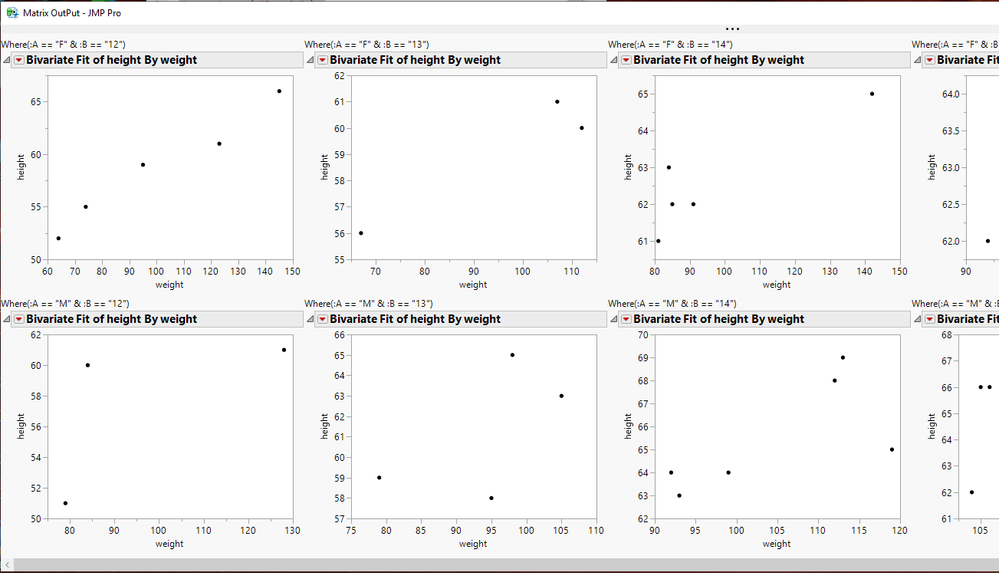Choose Language Hide Translation Bar
Highlighted

## Arrange unknown amount of charts

Hi,

I have 4 columns: X, Y, A, B.

X and Y are numeric, A and B are character.

I want to create multiple charts of X vs Y (by A and B), but arrange them differently than what I get by default.

What I get by default is all the charts in one long column.

What I want to get is rows by A and columns by B (sort of a matrix).

The thing is, that for each A there are different number of B's.

For example, for A=176 there are B=1...10, but for A=177 there are B=1...11.

So what I want is all A=176 charts on the first row, and all A=177 on the second row.

I guess my explanation is not great, so please see the two attached images of what I get and what I want.

Of course I want to know how to obtain this using JSL code.

Any help will be appreciated.

Thanks!

2 ACCEPTED SOLUTIONS

Accepted Solutions
Highlighted

## Re: Arrange unknown amount of charts

Hi Yotam,
That seems to be a perfect job for Graph Builder:
1) Drag your X column to the X Axis Box
2) Drag your Y Column to the Y Axis Box
3) Drag Column A to the Group X Box
4) Drag Column B to the Group Y Box
Let me know it this works for you.
Best,
TS
Thierry R. Sornasse
Highlighted

## Re: Arrange unknown amount of charts

Here is how I would do it.  There is an item I did in the JSL that were not required, but made the display look better.  I used the Substitute function to make the Where clause more readable.``````Names Default To Here( 1 );
dt = Open( "\$SAMPLE_DATA/Big Class.jmp" );
dt << set name( "Example" );
dt:age << set name( "B" ) << data type( character );
dt:sex << set name( "A" );
dt << delete columns( "name" );

Summarize( dt, aList = by( :A ) );
Summarize( dt, bList = by( :B ) );

NW = New Window( "Matrix OutPut", vlb = V List Box() );

For( i = 1, i <= N Items( aList ), i++,
hlb = H List Box();
For( k = 1, k <= N Items( bList ), k++,
If( N Rows( dt << get rows where( :A == alist[i] & :B == bList[k] ) ) > 0,
hlb << append(
V List Box(
Eval(
Substitute(
Expr(
biv = Bivariate(
Y( :height ),
X( :weight ),
Where( :A == __aList__ & :B == __bList__ )
)
),
Expr( __aList__ ), aList[i],
Expr( __bList__ ), bList[k]
)
)
)
)
)
);
vlb << append( hlb );
);``````
Jim
4 REPLIES 4
Highlighted

## Re: Arrange unknown amount of charts

Hi Yotam,
That seems to be a perfect job for Graph Builder:
1) Drag your X column to the X Axis Box
2) Drag your Y Column to the Y Axis Box
3) Drag Column A to the Group X Box
4) Drag Column B to the Group Y Box
Let me know it this works for you.
Best,
TS
Thierry R. Sornasse
Highlighted

## Re: Arrange unknown amount of charts

I haven't even considered graph builder, but it actually works great here.

Thanks!

Highlighted

## Re: Arrange unknown amount of charts

Here is how I would do it.  There is an item I did in the JSL that were not required, but made the display look better.  I used the Substitute function to make the Where clause more readable.``````Names Default To Here( 1 );
dt = Open( "\$SAMPLE_DATA/Big Class.jmp" );
dt << set name( "Example" );
dt:age << set name( "B" ) << data type( character );
dt:sex << set name( "A" );
dt << delete columns( "name" );

Summarize( dt, aList = by( :A ) );
Summarize( dt, bList = by( :B ) );

NW = New Window( "Matrix OutPut", vlb = V List Box() );

For( i = 1, i <= N Items( aList ), i++,
hlb = H List Box();
For( k = 1, k <= N Items( bList ), k++,
If( N Rows( dt << get rows where( :A == alist[i] & :B == bList[k] ) ) > 0,
hlb << append(
V List Box(
Eval(
Substitute(
Expr(
biv = Bivariate(
Y( :height ),
X( :weight ),
Where( :A == __aList__ & :B == __bList__ )
)
),
Expr( __aList__ ), aList[i],
Expr( __bList__ ), bList[k]
)
)
)
)
)
);
vlb << append( hlb );
);``````
Jim
Highlighted

## Re: Arrange unknown amount of charts

This is exactly what I was looking for. Thanks!

Would the code be different if B would also be numeric? For example in Big Class, B would be Age, so it could be both numeric and character.

I'm asking because when B is a character, it arranges the charts in the following order: 1,10,11,2,3...

While when B is numeric is arranges it 1,2,3...10,11 (which is more suited here).

Thanks!

Article Labels